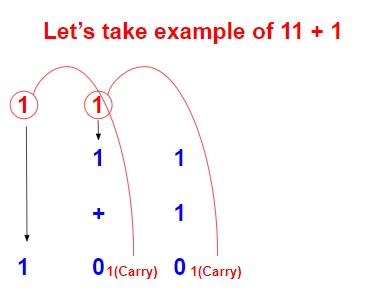# Program to add two binary strings in C++

Given two strings with binary number, we have to find the result obtained by adding those two binary strings and return the result as a binary string.

Binary numbers are those numbers which are expressed either as 0 or 1. While adding 2 binary numbers there is binary addition rule which is to be taken care of.

0+0 → 0
0+1 → 1
1+0 → 1
1+1 → 0, carry 1Input

str1 = {“11”}, str2 = {“1”}

Output

“100”

Input

str1 = {“110”}, str2 = {“1”}

Output

“111”

## Approach used below is as follows to solve the problem

• Traverse both the string from last

• Add the binary of two numbers

• If there are two 1’s then make it zero and carry 1.

• Return the result.

## Algorithm

Start
Step 1→ declare function to add two strings
set string result = ""
set int temp = 0
set int size_a = a.size() – 1
set int size_b = b.size() – 1
While (size_a >= 0 || size_b >= 0 || temp == 1)
Set temp += ((size_a >= 0)? a[size_a] - '0': 0)
Set temp += ((size_b >= 0)? b[size_b] - '0': 0)
Calculate result = char(temp % 2 + '0') + result
Set temp /= 2
Set size_a—
Set size_b—
End
return result
Step 2→ In main()
Declare string a = "10101", b="11100"
Stop

## Example

Live Demo

#include<bits/stdc++.h>
using namespace std;
string result = "";
int temp = 0;
int size_a = a.size() - 1;
int size_b = b.size() - 1;
while (size_a >= 0 || size_b >= 0 || temp == 1){
temp += ((size_a >= 0)? a[size_a] - '0': 0);
temp += ((size_b >= 0)? b[size_b] - '0': 0);
result = char(temp % 2 + '0') + result;
temp /= 2;
size_a--; size_b--;
}
return result;
}
int main(){
string a = "10101", b="11100";
cout<<"sum of strings are : "<<add(a, b);
return 0;
}

## Output

If run the above code it will generate the following output −

sum of strings are : 110001